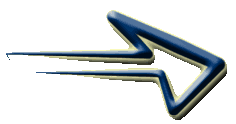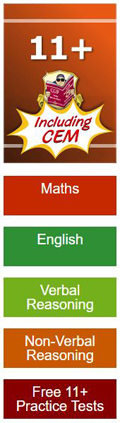Type 8 - 'Nets'

With this type of question you are given an image of a 3D cube on which you can see symbols/figures on three of the faces. To the right of the cube are five images of 'nets'
labelled 'a' to 'e' containing symbols/figures which are the possible answers.

You are required to determine which of the nets could be folded to make the 3D cube.

It is important that your child understands the concept of 3D Shapes and Nets before attempting this. Work through Shape and Space - 3D Shapes - Nets first. You may also find this 'Nets of a Cube' sheet useful to print, cut out and make.

Remember that the figures and diagrams consist of lines, shapes and shading/patterns. The shapes can shift in various ways. (rotate, reflect and transpose). The shapes can change in many ways. (increase/decrease, add and copy). They can consist of a movement, size or number sequence.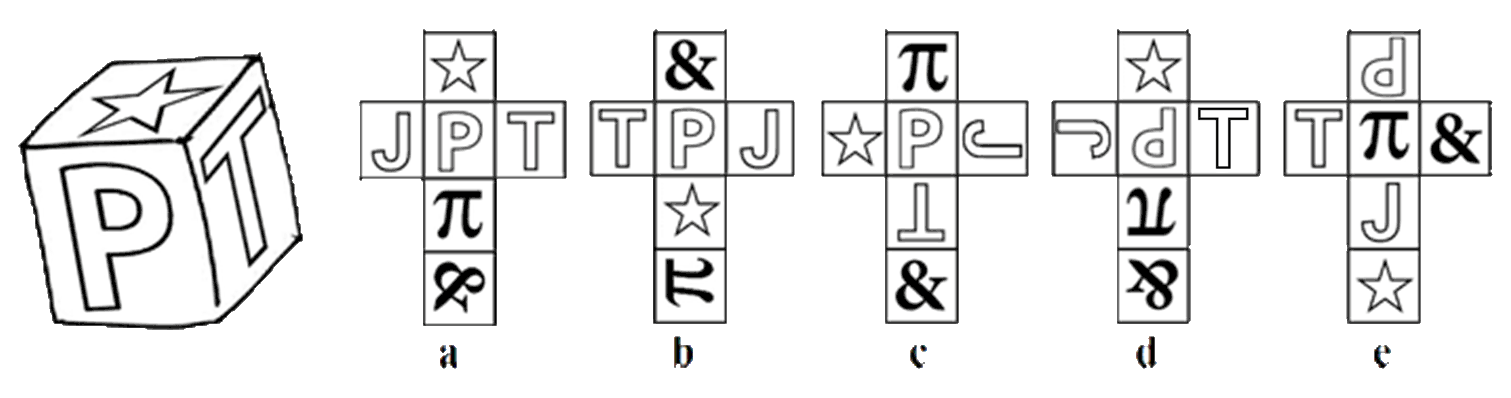Begin by looking at the three faces of the cube. Find an image that has an order of rotational symmetry of 1, in other words can only face one way, such as the 'P' in this example and imagine unfolding these three faces to form a net.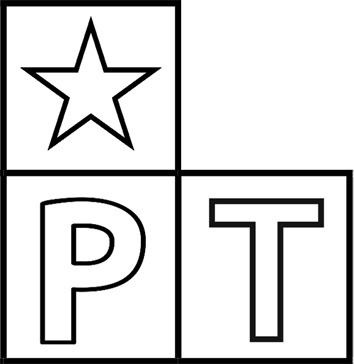You can see that the 'T' will be on the right of the 'P' with the star shape directly above either the 'P' or the 'T'.

Notice however how the position of the points of the star changes depending on which position it is in. When above the 'P' it has two points along the base with one at the top and when above the 'T', two points are along the left with one point on the right.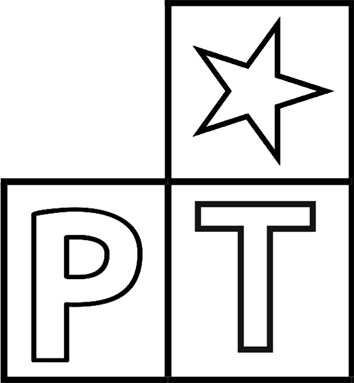Now compare this portion of a net with the nets labelled 'a' to 'e'

You can see that net 'a' is the only net that has the 'P' below the star and to the left of the 'T' and the points of the star are correct.

Unfortunately the nets are not always so straightforward as there are eleven different shapes of nets that can make a cube (see 3D Shapes - Nets and Nets of a Cube) but by doing this you can usually eliminate some of the options.

If possible, concentrate on the relationship between two adjacent faces with no rotational symmetry and check this with each net in turn and eliminate nets as you go.

Some children (and adults) find visualising a 2D net as a 3D cube very difficult. Try printing the questions, cutting out the nets and actually making the cubes.

Practice working with Non Verbal Reasoning Type 8 questions with this
Non Verbal Reasoning Type 8 'Nets' worksheet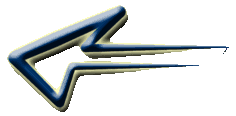Non Verbal Type 8 'Nets' worksheet